# School club

Six boys and nine girls and a teacher played in the school club. What is the probability that they will point at the boy when they reproach?

p =  0.375

### Step-by-step explanation:

$p=\frac{6}{6+9+1}=\frac{3}{8}=0.375$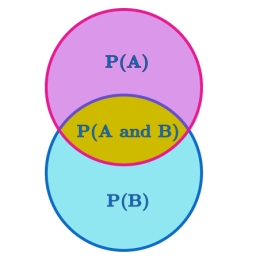Did you find an error or inaccuracy? Feel free to write us. Thank you!Tips to related online calculators
Would you like to compute count of combinations?

## Related math problems and questions:

• Two prizes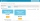In a class of 15 pupils (7 boys and 8 girls), Teacher Mae is giving away a random prize. What is probability that both prizes are won by girls?
• ClassroomOf the 26 pupils in the classroom, 12 boys and 14 girls, four representatives are picked to the odds of being: a) all the girls b) three girls and one boy c) there will be at least two boys
• Today in schoolThere are 9 girls and 11 boys in the class today. What is the probability that Suzan will go to the board today?
• Created trioWhat is the probability that in the created trio, which consists of 19 boys and 12 girls, they will be: a) the boys themselves b) the girls themselves c) 2 boys and 1 girl?
• Boys and girlsThere are eight boys and nine girls in the class. There were six children on the trip from this class. What is the probability that left a) only boys b) just two boys
• Boys and girlsThere are 11 boys and 18 girls in the classroom. Three pupils will answer. What is the probability that two boys will be among them?
• Class pairs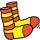In a class of 34 students, including 14 boys and 20 girls. How many couples (heterosexual, boy-girl) we can create? By what formula?
• Boys and girlsThere are 48 children in the sports club, boys are 10 more than girls. How many girls go to the club?
• Birth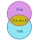Let's assume that the probability of the birth of a boy and a girl in the family is the same. What is the probability that in a family with five children, the youngest and oldest child is a boy?
• Boys and girls 2The ratio of boys to girls in math club is 4:3 . After 8 more girls joined the Club, the ratio become 1:1. How many members are there in the club now?
• School parliament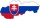There are 18 boys and 14 girls in the class. In how many ways can 3 representatives be elected to the school parliament, if these are to be: a) the boys themselves b) one boy and two girls
• Three dice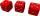What is the probability that when we roll three dice, we roll the numbers 1,2,3?
• Event probability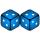The probability of event P in 8 independent experiments is 0.33. What is the probability that the event P occurs in one experiment (chance is the same)?
• Ace or king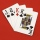What is the probability that we will choose an ace or a king when choosing from a deck of sevens cards?
• Study results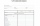There are 7 students in the class with excellent results, 6 praiseworthy, 5 good, 4 sufficient, and 3 insufficient students. What is the probability that it will be a good student when summoned?
• Six on diceWhat is the probability that when throwing two dice will fall at least one six?
• Sum 10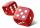What is the probability that two dice throw twice in a row will result the sum of 10?